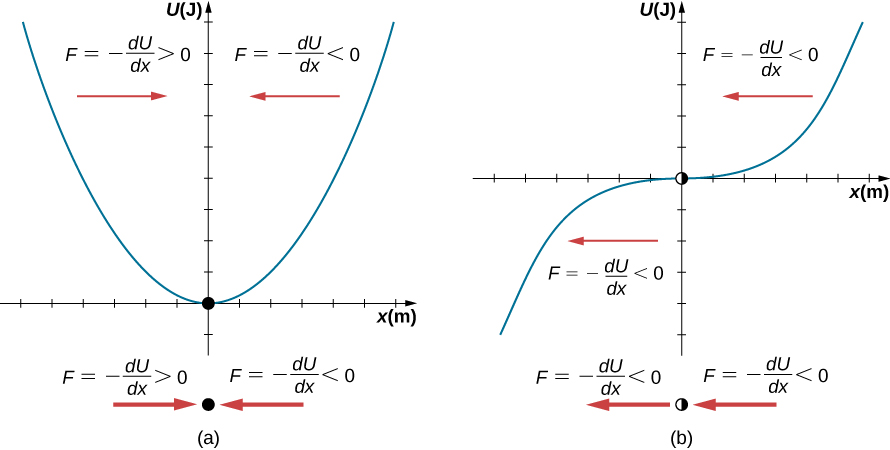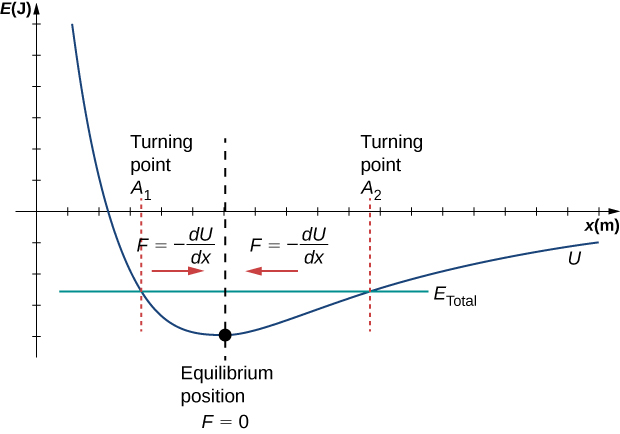# 15.2 Energy in simple harmonic motion  (Page 4/8)

 Page 4 / 8Two examples of a potential energy function. The force at a position is equal to the negative of the slope of the graph at that position. (a) A potential energy function with a stable equilibrium point. (b) A potential energy function with an unstable equilibrium point. This point is sometimes called half-stable because the force on one side points toward the fixed point.

A practical application of the concept of stable equilibrium points is the force between two neutral atoms in a molecule. If two molecules are in close proximity, separated by a few atomic diameters, they can experience an attractive force. If the molecules move close enough so that the electron shells of the other electrons overlap, the force between the molecules becomes repulsive. The attractive force between the two atoms may cause the atoms to form a molecule. The force between the two molecules is not a linear force and cannot be modeled simply as two masses separated by a spring, but the atoms of the molecule can oscillate around an equilibrium point when displaced a small amount from the equilibrium position. The atoms oscillate due the attractive force and repulsive force between the two atoms.

Consider one example of the interaction between two atoms known as the van Der Waals interaction. It is beyond the scope of this chapter to discuss in depth the interactions of the two atoms, but the oscillations of the atoms can be examined by considering one example of a model of the potential energy of the system. One suggestion to model the potential energy of this molecule is with the Lennard-Jones 6-12 potential :

$U\left(x\right)=4\epsilon \left[{\left(\frac{\sigma }{x}\right)}^{12}-{\left(\frac{\sigma }{x}\right)}^{6}\right].$

A graph of this function is shown in [link] . The two parameters $\epsilon$ and $\sigma$ are found experimentally.The Lennard-Jones potential energy function for a system of two neutral atoms. If the energy is below some maximum energy, the system oscillates near the equilibrium position between the two turning points.

From the graph, you can see that there is a potential energy well, which has some similarities to the potential energy well of the potential energy function of the simple harmonic oscillator discussed in [link] . The Lennard-Jones potential has a stable equilibrium point where the potential energy is minimum and the force on either side of the equilibrium point points toward equilibrium point. Note that unlike the simple harmonic oscillator, the potential well of the Lennard-Jones potential is not symmetric. This is due to the fact that the force between the atoms is not a Hooke’s law force and is not linear. The atoms can still oscillate around the equilibrium position ${x}_{\text{min}}$ because when $x<{x}_{\text{min}}$ , the force is positive; when $x>{x}_{\text{min}}$ , the force is negative. Notice that as x approaches zero, the slope is quite steep and negative, which means that the force is large and positive. This suggests that it takes a large force to try to push the atoms close together. As x becomes increasingly large, the slope becomes less steep and the force is smaller and negative. This suggests that if given a large enough energy, the atoms can be separated.

state the properties of a base
state 3 properties of a base
State three properties of a base
mubarak
a stone is projected from the top of the tower 24.5m high with velocity of 39.2m/s at an angle of 30° with horizontal compute time taken to hit he ground
it took a car driver 30mins to travel a distance of 50km.what is the average speed
Suppose the master cylinder in a hydraulic system is at a greater height than the cylinder it is controlling. Explain how this will affect the force produced at the cylinder that is being controlled.
Why is popo less than atmospheric? Why is popo greater than pipi?
Louise
The old rubber boot shown below has two leaks. To what maximum height can the water squirt from Leak 1? How does the velocity of water emerging from Leak 2 differ from that of Leak 1? Explain your responses in terms of energy.
Louise
David rolled down the window on his car while driving on the freeway. An empty plastic bag on the floor promptly flew out the window. Explain why.
the pressure differential exerted a force on the bag greater than the gravitational force holding it on the floor.
gtitboi
what is angular velocity
The rate of change in angular displacement is defined as angular velocity.
Manorama
a length of copper wire was measured to be 50m with an uncertainty of 1cm, the thickness of the wire was measured to be 1mm with an uncertainty of 0.01mm, using a micrometer screw gauge, calculate the of copper wire used
Mustapha
it took a car driver 30 mins to travel a distance of 50km what is the average speed .
Isiguzo
If centripetal force is directed towards the center,why do you feel that you're thrown away from the center as a car goes around a curve? Explain
if there is a centripetal force it means that there's also a centripetal acceleration, getting back to your question, just imagine what happens if you pull out of a car when it's quickly moving or when you try to stop when you are running fast, anyway, we notice that there's always a certain force..
Lindomar
... that tends to fight for its previous direction when you try to attribute to it an opposite one ou try to stop it.The same thing also happens whe a car goes around a curve, the car it self is designed to a"straight line"(look at the position of its tyres, mainly the back side ones), so...
Lindomar
... whenever it goes around a curve, it tends to throw away its the occupiers, it's given to the fact that it must interrupt its initial direction and take a new one.
Lindomar
Which kind of wave does wind form
calculate the distance you will travel if you mantain an average speed of 10N m/s for 40 second
400m/s
Feng
hw to calculate the momentum of the 2000.0 elephant change hunter at a speed of 7.50 m/s
how many cm makes 1 inches
2.5
omwoyo
2.54cm=1inche
omwoyo
how do we convert from m/s to km/hr
When paddling a canoe upstream, it is wisest to travel as near to the shore as possible. When canoeing downstream, it may be best to stay near the middle. Explain why?
Explain why polarization does not occur in sound
one ship sailing east with a speed of 7.5m/s passes a certain point at 8am and a second ship sailing north at the same speed passed the same point at 9.30am at what distance are they closet together and what is the distance between them then

#### Get Jobilize Job Search Mobile App in your pocket Now!By RhodesBy Sarah WarrenBy OpenStaxBy OpenStaxBy OpenStaxBy Jemekia WeedenBy Stephanie RedfernBy Brooke DelaneyBy Brooke DelaneyBy Rohini Ajay